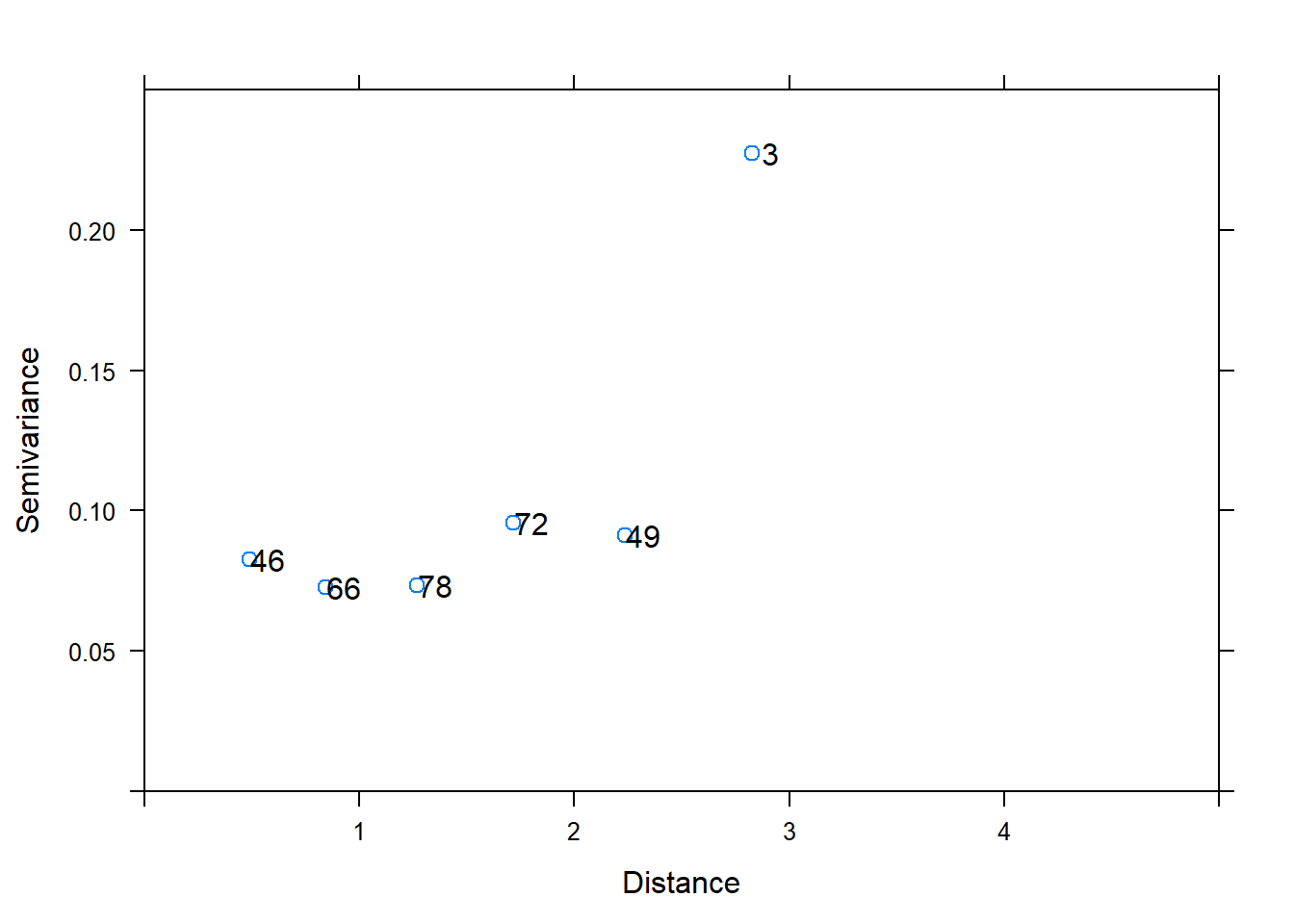## R Markdown for Duke Farms - Grassland Field (skeet shoot field)

The data is available HERE.

library(gstat)

### Part 1: When ‘n = 150’

##### 1.1 Compute undirected (isotropic) empirical semivariogram
var1 <- variogram(Total_C ~ 1, ~x.axis + y.axis, cutoff = 500, width = 30, data = mc)

# create figure of empirical semivariogram
plot(var1, plot.numbers = TRUE, xlab = "Distance", ylab = "Semivariance", cex = 1, cex.axis = 2, xlim=c(0, 550), ylim=c(0.0, 0.25))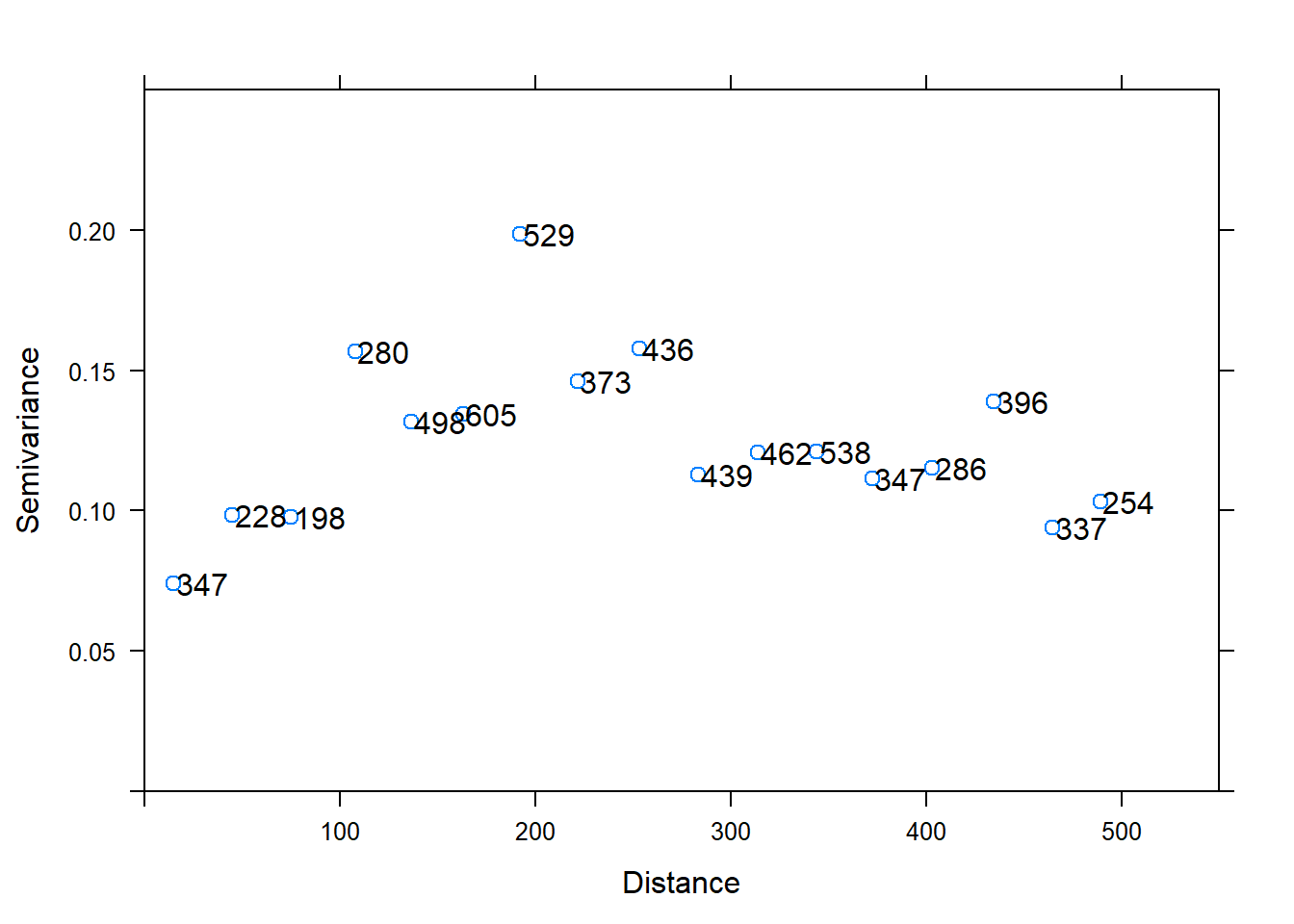#### Figure 1: Undirected semivariogram of Total_C .

##### 1.1.2 Semivariogram models

To fit theoretical semivariograms, the function ‘fit.variogram’ of the package ‘gstat’ is used. Sill, nugget and range are set to be calculated based on the empirical variogram data, which is also used to fit the model ‘(fit.method=1)’. This method fits the variogram model to the experimental variogram, using weighted least squares with weight = Nj, where Nj is the number of observations in the j -th distance class (bin) (from: http://www.gstat. org/gstat.pdf, Table 4.2). The exponential model is used here. Semivariogram models are only fitted to undirected empirical semivariograms as the number of point pairs per bin in the directed ones is very low and predictions therefore have less power (the number of points per bin (np) for the undirected semivariograms is as high as for all directed semivariograms together).

##### compute semivariogram model based on the empiral semivariogram ‘var1’**
mod1 <- fit.variogram(var1, vgm(psill = NA, "Exp", range = NA, 1), fit.sills = TRUE, fit.ranges = TRUE, fit.method = 1)
## Warning in fit.variogram(var1, vgm(psill = NA, "Exp", range = NA, 1), fit.sills
## = TRUE, : No convergence after 200 iterations: try different initial values?

##### show nugget, psill and range of the semivariogram model
mod1
##   model      psill    range
## 1   Nug 0.04865517  0.00000
## 2   Exp 0.08499639 40.38164

##### show the (weighted) sum of squared errors of the fitted model
attr(mod1, "SSErr")
##  4.346393

##### plot figure of semivariogram model
plot(var1, plot.numbers = TRUE, model = mod1, xlab = "Distance", ylab = "Semivariance", xlim=c(0, 550), ylim=c(0.0, 0.25))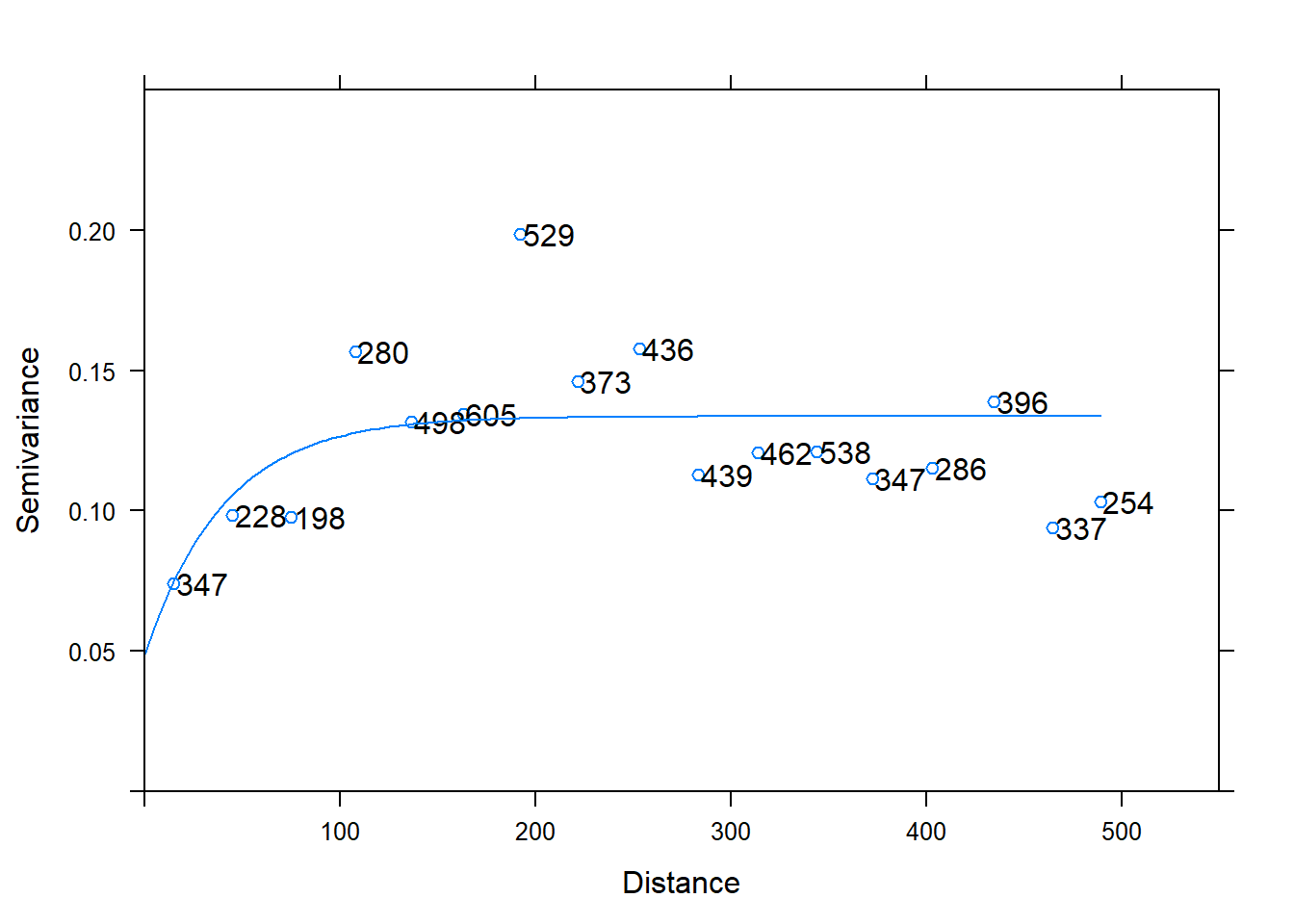### Part 2: when ‘n = 94’ (I removed the data from 0.5-m sampling next)

# select all data where the distance is not (! = is not) equal to 0.5

mc1 = subset(mc, !distance== "0.5")

##### 2.1 Compute undirected (isotropic) empirical semivariogram
var1 <- variogram(Total_C ~ 1, ~x.axis + y.axis, cutoff = 500, width = 30, data = mc1)
#
#
# create figure of empirical semivariogram
plot(var1, plot.numbers = TRUE, xlab = "Distance", ylab = "Semivariance", cex = 1, cex.axis = 2, xlim=c(0, 550), ylim=c(0.0, 0.25))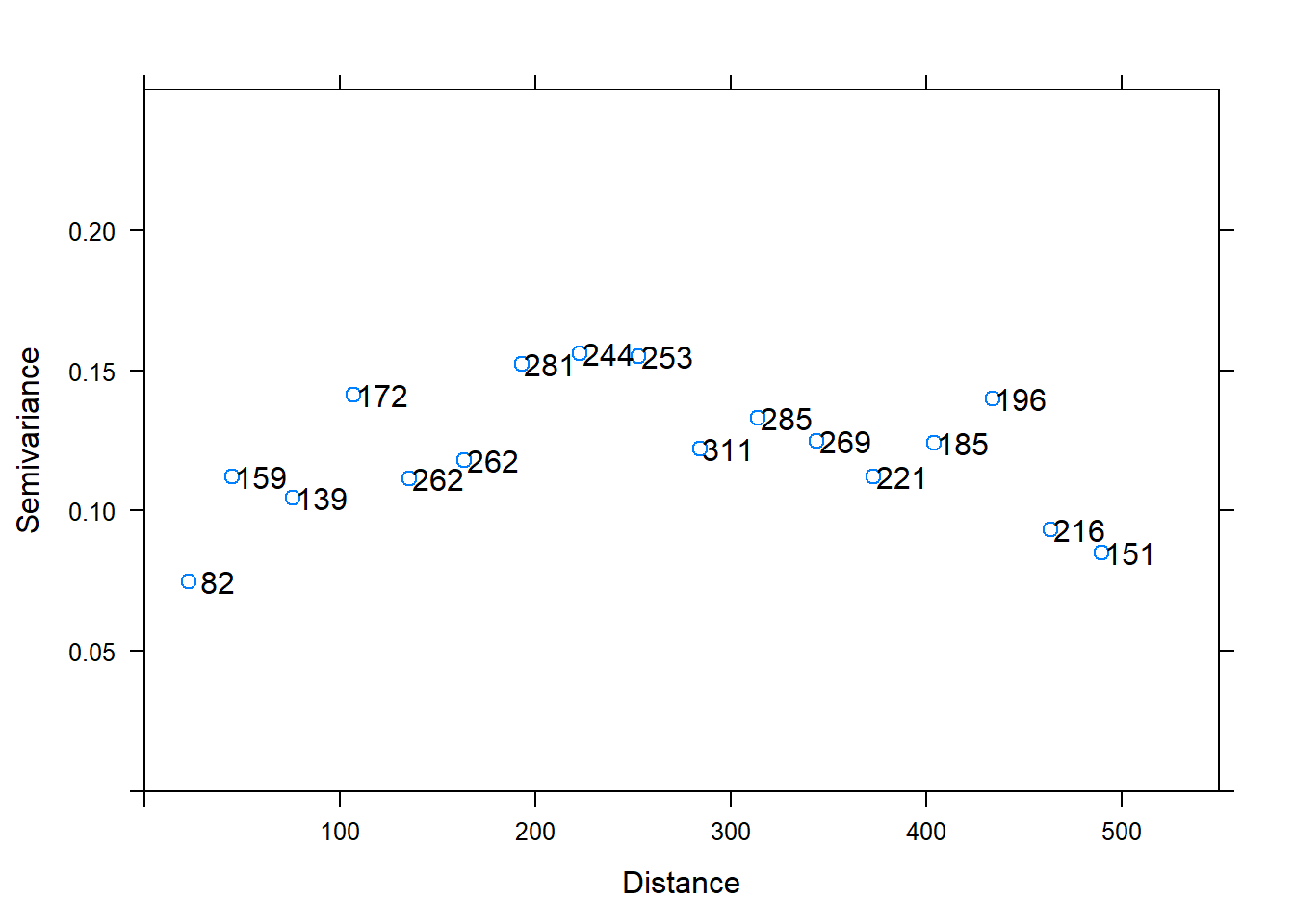#### Figure 3: Undirected semivariogram of Total_C . The empirical semivariogram shows a spatial distribution without the 0.5-m samples.

##### 2.1.2 Semivariogram models

Now we fit theoretical semivariograms to those empirical semivariograms which showed an autocorrelation structure in the previous data analyses.

##### compute semivariogram model based on the empiral semivariogram ‘var1’
mod1 <- fit.variogram(var1, vgm(psill = NA, "Exp", range = NA, 1), fit.sills = TRUE, fit.ranges = TRUE, fit.method = 1)

##### show nugget, psill and range of the semivariogram model
mod1
##   model      psill    range
## 1   Nug 0.01721994  0.00000
## 2   Exp 0.11024465 28.24095

##### show the (weighted) sum of squared errors of the fitted model
attr(mod1, "SSErr")
##  1.364782

##### plot figure of semivariogram model
plot(var1, plot.numbers = TRUE, model = mod1, xlab = "Distance", ylab = "Semivariance", xlim=c(0, 550), ylim=c(0.0, 0.25))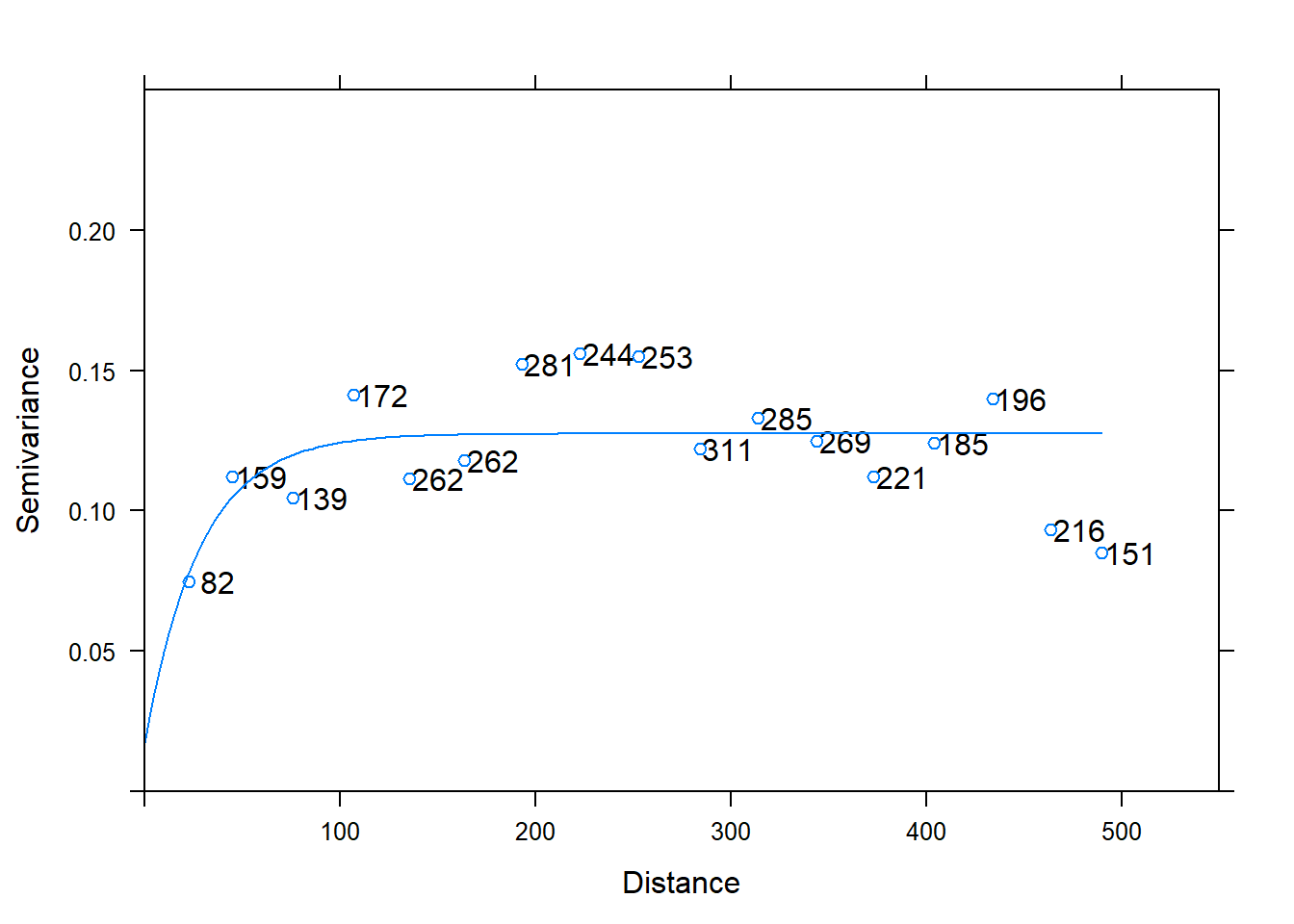#### Figure 4: Exponential model fit on Total_C.

##### 3.1 Compute undirected (isotropic) empirical semivariogram
library(gstat)
##  "SpatialPointsDataFrame"
## attr(,"package")
##  "sp"
var1 <- variogram(z ~ 1, ~x.axis + y.axis, cutoff = 10, width = 0.5, data = JY)

# create figure of empirical semivariogram
plot(var1, plot.numbers = TRUE, xlab = "Distance", ylab = "Semivariance", cex = 1, cex.axis = 2, xlim=c(0, 5), ylim=c(0.0, 0.25))